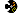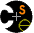CSE326 Winter 2006

 ProjectsProject 1Project 2 - phase AProject 2 - phase BProject 3 HomeworkHomework 1Homework 2Homework 3 AdministrativeHomeMessage BoardAnnoucement ArchiveClass List ArchiveAnonymous FeedbackMail Instructor & TAs LecturesCalendar & Slides HandoutsFirst Day Handout PoliciesGeneral GuidelinesGrading PoliciesProgramming GuidelinesWriteup Guidelines ComputingUnix Basics# Homework 1

## Asymptotic Analysis and Priority Queues Due: Wednesday, Jan 25, at the beginning of class

This assignment is designed to give you practice with the mathematical concepts behind algorithmic analysis as well as to introduce working with various implementations of priority queues, which will be useful in the next programming project.

Note: This is a written assignment, so late homework will not be accepted. There is no electronic turn-in. Problem numbers refer to the Weiss textbook.

Total: 80 points.

1)      [Basic math] (10 points) Problem 1.8, parts (a), (b)

2)      [Big-O notation] (10 points) Simplify the following expressions as much as possible using the Big-O notation, expressing each as big-O of some function of n, e.g. 3 n + log n = O(n). You don't need to give the constants c and n0 for this problem, but for practice, try finding them for some of your answers.

`  a. n3 + 100 n`
` `
`  b. 2 n2 - n + 2100`
` `
`  c. 3 log log n + log2 n`
` `
`  d. 10 n2 - 5 n + n3 log n`
` `
`  e. 2.5n + n100`

3)      [Program analysis] (10 points) Problem 2.7, part (a) only, fragments (2) to (6). Briefly justify your answer.

4)      [Recursive equations] (5 points) Estimate the running time of the following function using the Big-O notation. Make sure to state the recursive equation for running time, and solve it using your favorite method (such as expansion, induction).

` `
`int fun_a(int n) {`
`  if (n<=1) return 100;`
`  else return 2*fun_a(n-1);`
`}`
` `

5)      [Binary Min Heaps] (5 points total)

a)      Show the result of inserting: 10,12,1,14,6,5,8,15,3,9,7,4,11,13,2 one at a time into an initially empty  binary heap. Check your work!

b)      Show the result of performing two deleteMin operations on the heap you created in part a).

6)      [d-Heaps] (10 points) Problem 6.14. Also indicate the index of the array that contains (a) the root, (b) the next available location for insert. (Remember the root is stored in position 1)

7)      [Leftist Heaps] (10 points) Problem 6.19

8)      [Skew Heaps] (10 points) Problem 6.27. Show the result for the intermediate skew heap resulting after adding 1-3 and 1-6, and the final heap after adding 1-15. (You may show all 15 heaps if it's easier; although we will look at only the above 3 for grading.)

9)      [Binomial Qs] (10 points) Problem 6.32Computer Science & Engineering University of Washington Box 352350 Seattle, WA  98195-2350 (206) 543-1695 voice, (206) 543-2969 FAX [comments to cary]